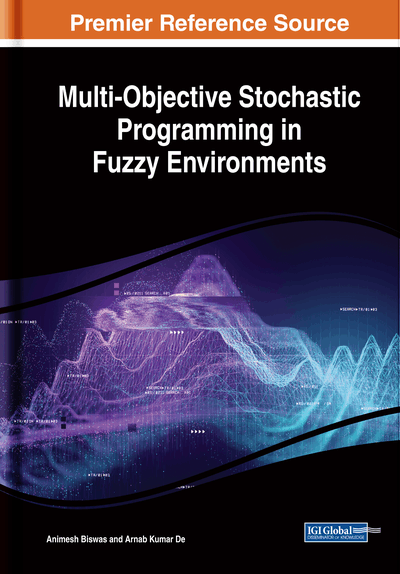# Methodology for Solving Multi-Objective Quadratic Programming Problems in a Fuzzy Stochastic Environment

DOI: 10.4018/978-1-5225-8301-1.ch005
Available
\$33.75
List Price: \$37.50
10% Discount:-\$3.75
TOTAL SAVINGS: \$3.75

## Abstract

This chapter presents two methodologies for solving quadratic programming problems with multiple objectives under fuzzy stochastic environments. The right side parameters of the chance constraints of both the models are chosen as fuzzy random variables (FRVs) following different probability distributions. Like the previous chapters, chance constrained programming (CCP) methodology is employed to the fuzzy chance constraints to develop fuzzy programming model. In the first model, cut of fuzzy sets and fuzzy partial order relations are incorporated to the fuzzy programming model to develop an equivalent deterministic model. For the second model, defuzzification method of fuzzy numbers (FNs), which are presented in Chapter 2, are taken into consideration to generate equivalent quadratic programming model in a crisp environment. As the objective functions are quadratic in nature, it is easy to understand that the membership functions obtained through methodological development process are also quadratic in nature. To linearize the quadratic membership functions, linearization techniques are employed in this chapter. Finally, for achieving the maximum degree of each of the membership goals of the objectives, a fuzzy goal programming (FGP) approach is developed for the linearized membership goals and solved by minimizing under-deviational variables and satisfying modified system constraints in fuzzy stochastic decision-making environments. To illustrate the acceptability of the developed methodology presented in this chapter, some numerical examples are included.
Chapter Preview
Top

## 5.1 Quadratic Programming Model In Fuzzy Stochastic Environment

In single objective mathematical programming model only one objective is considered subject to some set of system constraints. Most of the times this type of mathematical programming models fails to represent practical situations properly as real life decision making problem in various fields of science, engineering and management etc., involve multiple conflicting objectives. Mathematical programming problems with multiple objectives are termed as multi-objective programming. The authors throughout this book discuss different types of multi-objective programming problems. Hwang and Masud (1979) classified the methods for solving multi-objective optimization problems into three categories, viz., priori methods, interactive methods and posteriori methods. In the literature, a variety of algorithms (Chen and Chou, 1996; Gass and Roy, 2003; Ida, 2005; Li et al., 2006) for finding efficient solutions to multi-objective optimization problems were proposed. Also, in multi-objective environments some additional preference information is needed from the decision makers (DMs) for getting better solution from a set of efficient solutions. Again, it is found that the objectives of various practical decision making problems may not be represented by linear functions, these objectives includes some non-linear natures, especially, quadratic natures. To handle such mathematical programming problems the authors present some methodology to solve quadratic programming problems. Multi-objective quadratic programming is considered as a special case of non-linear programming in which either the objectives or constraints or both are quadratic in nature. Korhonen and Yu (1997) discussed a reference direction approach to solve multi-objective quadratic programming problems. Ammar and Khalifa (2003) studied quadratic programming to solve portfolio optimization problems. In 2014, Brito et al. (2014) proposed a new approach for multi-objective quadratic optimization. In recent years, Fliege and Vaz (2016) and Ansary and Panda (2018) developed a methodology for solving multi-objective quadratic programming problems based on sequential quadratic programming technique. Syaripuddin et al. (2017) extended Wolfe’s method for solving quadratic programming problems by taking model parameters as interval coefficients.

In the preceding chapters the authors already mentioned that in conventional linear programming model it is necessary that the parameters are known precisely. But real world context, however, the parameters are seldom known exactly and, hence, have to be estimated. Therefore, some kind of uncertainty may involve with these problems. These uncertain parameters may be possibilistic or probabilistic in nature. Also some situation arises when these uncertain parameters are represented as a combination of both possibilistic and probabilistic uncertainties. The context that arises due to the simultaneous presence of randomness and fuzziness is known as hybrid fuzzy environment.

The possibilistic uncertainty involved inherently with the DMs’ ambiguous understanding of the nature of parameters associated with the problem. As stated earlier, fuzzy set theory, introduced by Zadeh (1965), are used to represent possibilistic uncertainties. Zimmermann (1978) showed that the solutions obtained by fuzzy linear programming are always efficient. Abo-Sinha (2001) discussed multi-objective optimization for solving non-linear multi-objective bi-level programming problems in fuzzy environment. Aggarwal and Sharma (2013) proposed a procedure for solving non-convex multi-objective quadratic programming under fuzzy environment. Gabr (2015) analyzed quadratic and non-linear programming models in fully fuzzy environment. Recently, Khalifa (2017) studied a multi- objective quadratic programming problem with possibilistic variables coefficients matrix in the objective functions.

## Complete Chapter List

Search this Book:
Reset